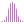#LinRegSlope (MDX)

Calculates the linear regression of a set, and returns the value of the slope in the regression line, y = ax + b.

#### Syntax

LinRegSlope( <List> , , )